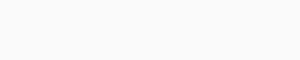Asian Science and Maths Olympiad (ASMO) is a competition platform designed to challenge and evaluate student’s knowledge in Mathematics and Science at their grade level. The questions in the Olympiad will stretch their knowledge and understanding of the concepts. Our syllabus fits nicely into the syllabus that concentrates on non-routine problem-solution to prepare the students for the competition. With the expansion of STEM education worldwide, ASMO certainly answers the need of it. Students will be well prepared with the skills to meet the science and technology challenges.

In Malaysia, ASMO is officially endorsed by Ministry of Education and all participants will obtain curriculum marks. In 2018 alone, Asian Science and Mathematics Olympiad has received 70,000 entries from across the ASEAN countries. We are targeting for the number to increase at 80,000 for 2019.

We are also proud to present that ASMO International is a new effort by ASMO Malaysia which started in 2017 in Pattaya, Thailand. When it was initially launched, the competition was setup via collaboration with ASMOPSS and ASMO Thai was the host for the competition. In 2018, Malaysia has become the host for the competition and it was participated by 10 Asian countries.

The idea of opening up a new competition platform which is ASMO International is to expand the level of competition and to provide more opportunities for primary and secondary school students to experience international engagement. (sc : http://asmo2u.com/about-us)

Berikut ini problems and solution ASMO 2019 grade 6

1. Calculate：$$238÷238\frac{238}{239}=?$$

\begin{align} 238 ÷ 238\frac{238}{239}&= 238 ÷\frac{238×239+238}{239}\\ &= 238 ×\frac{239}{238×239+238}\\ &=\frac{238×239}{238×239+238}\\ &=\frac{238×239}{238(239+1)}\\ &=\frac{239}{239+1}\\ &=\frac{239}{240}\\ \end{align}

2. The sum of father’s and son’s age is 64 years old. Three times the son’s age is 8 years old older than father’s age. What are the ages of father and son respectively?

Umur Ayah : $$𝐵$$
Umur Anak : $$𝐴$$
$$𝐴 + 𝐵 = 64$$
$$𝐵 = 3𝐴 + 8$$
Subtitusi $$𝐵 = 3𝐴 + 8$$ ke persamaan $$𝐴 + 𝐵 = 64$$

$$𝐴 + 3𝐴 + 8 = 64$$
$$⇒4𝐴 = 64 − 8$$
$$⇒4𝐴 = 56$$
$$⇒𝐴 = 56 ∶ 4 = 14$$

Umur Ayah : $$64 − 14 = 50$$ 𝑡𝑎ℎ𝑢𝑛
Jadi umur Ayah adalah $$50$$ tahun dan umur anak adalah : $$14$$ tahun

3. The average value of A, B and C is 70. A has a value of 80 while B and C have the same value, what is the value of B?

$$\frac{𝐴 + 𝐵 + 𝐶}{3}= 70$$
$$⇒𝐴 + 𝐵 + 𝐶 = 210$$
$$⇒80 + 𝐵 + 𝐵 = 210$$
$$⇒80 + 2𝐵 = 210$$
$$⇒2𝐵 = 130$$
$$⇒𝐵 = 65$$

Jadi nilai $$B$$ adalah $$65$$

4. 150kg minus $$\frac{1}{6}$$ of its weight and $$\frac{1}{6}$$ kg. How much weight is remaining?

not yet available

5. Father has RM1500.00. He bought a table with 30% of his money. He bought a vacuum cleaner with 55% of the remaining money. Lastly, he gave half of the remaining money to mother. How much money did mother receive?

Membeli meja $$: \frac{30}{100}× 1500 = 𝑅𝑀450, tersisa :1500 – 450 = 1050$$
Membeli penyedot debu $$: \frac{55}{100}× 1050 = 𝑅𝑀577,5$$ , tersisa $$:1050 – 577,5 = 472,5$$
Uang yang diberikan ke Ibu $$:\frac{1}{2}× 𝑅𝑀472,5 = 𝑅𝑀236,25$$

6. The average value of 8 numbers is 180. After adding in 120 and 150, what is the new average value?

$$\frac{𝑥_1 + 𝑥_2 + 𝑥_3 + ⋯ + 𝑥_8}{8}= 180$$
$$𝑥_1 + 𝑥_2 + 𝑥_3 + ⋯ + 𝑥_8 = 1440$$

Tambah dua bilangan lagi yaitu $$120$$ dan $$150$$

$$\frac{𝑥_1 + 𝑥_2 + 𝑥_3 + ⋯ + 𝑥_8 + 120 + 150}{10}=\frac{1440 + 120 + 150}{10}=\frac{1710}{10}=171$$

Jadi rata-rata barunya adalah $$171$$

7. The current age for little brother is $$\frac{1}{2}$$ of big brother’s age. 9 years ago, little brother’s age is only $$\frac{1}{5}$$ of big brother’s age. How old is big brother now?

Misalkan umur kakak adalah $$K$$ dan umur Adik adalah $$A$$

$$𝐴 =\frac{1}{2}$$
$$𝐾 ⇒ 𝐾 = 2𝐴$$

$$9$$ tahun yang lalu umur adik hanya $$\frac{1}{5}$$ dari umur kakak

$$𝐴 − 9 =\frac{1}{5}(𝐾 − 9)$$
$$5𝐴 − 45 = 𝐾 − 9$$

Ganti nilai $$K$$ dengan $$2A$$

$$5𝐴 − 45 = 2𝐴 − 9$$
$$5𝐴 − 2𝐴 = 45 − 9$$
$$3𝐴 = 36$$
$$𝐴 = 12$$

Jadi umur kakak sekarang adalah $$2(12)=24$$ tahun.

8. To cut a piece of wood into 4 sections requires 6 minutes. How long does it take to cut the piece of wood into 8 sections?

Untuk memotong kayu menjadi 4 bagian maka kayu dipotong sebanyak 3 kali, 3 kali membutuhkan 6 menit, 1 kali potongan membutuhkan waktu 2 menit.
Jadi untuk memotong kayu menjadi 8 bagian dibutuhkan 7 kali potongan, waktu yang diperlukan adalah 7 × 2 = 14 menit.

9. If someone invests in a stock that reduces in value for 20% in the first year. How many percent must the stock increase in value so that the stock value comes back to its original value?

Misalkan investasi awal $$100$$
Tahun pertama terjadi penurunan sebanyak $$20%$$ atau
$$\frac{20}{100}× 100 = 20$$. Modal Investasinya sekarang adalah $$80$$.
Tahun berikut dia menginginkan uangnya kembali semula, berapa persen keuntungan yangharus diperoleh?
Supaya uangnya kembali ke awal maka harus naik $$20$$.
$$\frac{20}{80}× 100\% = 25\%$$Luas satu persegi (alas) = $$20$$ cm², pada saat di satukan membentuk balok banyak persegi yang terlihat sebanyak $$10$$ persegi. Jadi luas permukaan balok adalah $$10 × 20 = 200$$𝑐𝑚²